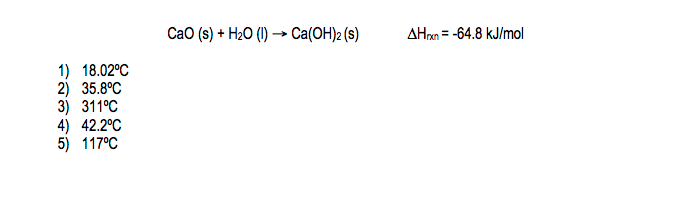# Problem: 10.1 g CaO is dropped into a Styrofoam coffee cup containing 157 g H2O at 18.0ºC. If the following reaction occurs, what temperature will the water reach, assuming that the cup is a perfect insulator and that the cup absorbs only a negligible amount of heat? (the specific heat of water = 4.18 J/gºC)

###### FREE Expert Solution
92% (423 ratings)
###### Problem Details

10.1 g CaO is dropped into a Styrofoam coffee cup containing 157 g H2O at 18.0ºC. If the following reaction occurs, what temperature will the water reach, assuming that the cup is a perfect insulator and that the cup absorbs only a negligible amount of heat? (the specific heat of water = 4.18 J/gºC)What scientific concept do you need to know in order to solve this problem?

Our tutors have indicated that to solve this problem you will need to apply the Thermochemical Equation concept. You can view video lessons to learn Thermochemical Equation. Or if you need more Thermochemical Equation practice, you can also practice Thermochemical Equation practice problems.

What is the difficulty of this problem?

Our tutors rated the difficulty of10.1 g CaO is dropped into a Styrofoam coffee cup containing...as high difficulty.

How long does this problem take to solve?

Our expert Chemistry tutor, Jules took 4 minutes and 52 seconds to solve this problem. You can follow their steps in the video explanation above.

What professor is this problem relevant for?

Based on our data, we think this problem is relevant for Professor Charlesworth's class at Michigan Technological University.